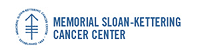## Memorial Sloan-Kettering Cancer Center, Dept. of Epidemiology & Biostatistics Working Paper Series

#### Abstract

The integrated likelihood (also called the marginal likelihood or the normalizing constant) is a central quantity in Bayesian model selection and model averaging. It is defined as the integral over the parameter space of the likelihood times the prior density. The Bayes factor for model comparison and Bayesian testing is a ratio of integrated likelihoods, and the model weights in Bayesian model averaging are proportional to the integrated likelihoods. We consider the estimation of the integrated likelihood from posterior simulation output, aiming at a generic method that uses only the likelihoods from the posterior simulation iterations. The key is the harmonic mean identity, which says that the reciprocal of the integrated likelihood is equal to the posterior harmonic mean of the likelihood. The simplest estimator based on the identity is thus the harmonic mean of the likelihoods. While this is an unbiased and simulation-consistent estimator, its reciprocal can have infinite variance and so it is unstable in general.

We describe two methods for stabilizing the harmonic mean estimator. In the first one, the parameter space is reduced in such a way that the modified estimator involves a harmonic mean of heavier-tailed densities, thus resulting in a finite variance estimator. The resulting estimator is stable. It is also self-monitoring, since it obeys the central limit theorem, and so confidence intervals are available. We discuss general conditions under which this reduction is applicable. The second method is based on the fact that the posterior distribution of the log-likelihood is approximately a gamma distribution. This leads to an estimator of the maximum achievable likelihood, and also an estimator of the effective number of parameters that is extremely simple to compute from the loglikelihoods, independent of the model parametrization, and always positive. This yields estimates of the log integrated likelihood, and posterior simulation-based analogues of the BIC and AIC model selection criteria, called BICM and AICM. We illustrate the proposed methods through several examples.

#### Disciplines

Statistical Methodology | Statistical Theory

COinS